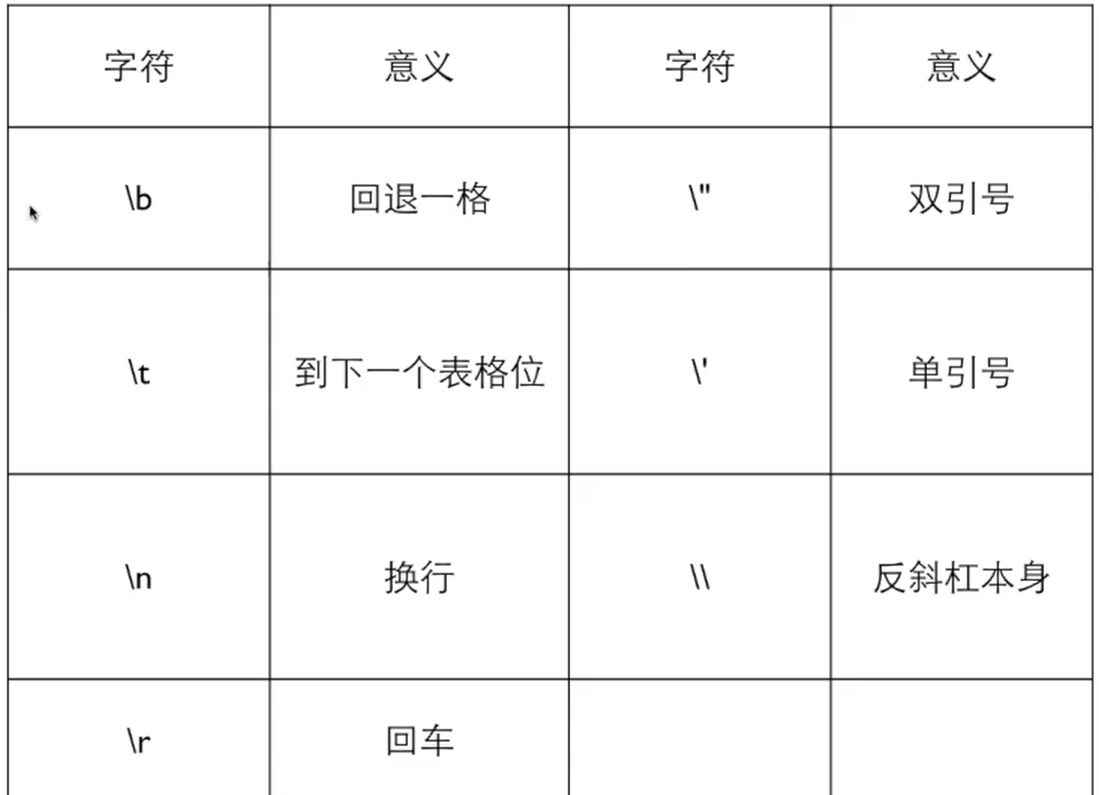##### 字符类型

char是一种整数，也是一种特殊的类型：字符。 可以用单引号''表示字符的字面量，如字符'a'和字符'1'。 在printf和scanf里可以使用%c做字符的输入输出。

###### 字符的输入输出

char类型的变量c，c不仅可以是一个整数，还可以是一个字符。 判断整数1和字符'1'是否相等：

``````#include <stdio.h>

int main()
{
char a;
char b;
a = 1;
b = '1';
if (a == b){
printf("相等");
} else{
printf("不相等");
}
return 0;
}``````
``````不相等
--------------------------------
Process exited after 0.01181 seconds with return value 0``````

``````#include <stdio.h>

int main()
{
char a;
char b;
a = 1;
b = '1';
printf("a=%d\n",a);
printf("b=%d\n",b);
return 0;
}``````
``````a=1
b=49

--------------------------------
Process exited after 0.01567 seconds with return value 0``````

###### 输入字符'1'给char a

::: tip C语言中，%c表示按照字符输出；%d表示按照整形输出。 ::: 使用scanf("%c",&a); ——>1或者scanf("%d",&a) ——>49： scanf使用%c读取输入值：

``````#include <stdio.h>

int main()
{
char a;
scanf("%c",&a);  //scanf使用%c读取a
printf("a=%d\n",a);  //当a是一个整数时输出a的整数值
printf("a='%c'\n",a);  //当a是一个字符时输出字符a的值
return 0;
}``````
``````1
a=49
a='1'

--------------------------------
Process exited after 9.881 seconds with return value 0``````

scanf使用%d读取输入值：

``````#include <stdio.h>

int main()
{
int i;
char a;
scanf("%d",&i);  //scanf使用%d来读取
a = i;
printf("a=%d\n",a);
printf("a='%c'\n",a);
return 0;
}``````
``````49
a=49
a='1'

--------------------------------
Process exited after 1.866 seconds with return value 0``````

``````#include <stdio.h>

int main()
{
if( 49 == '1' ){
printf("ok");
}
return 0;
}``````
``````ok
--------------------------------
Process exited after 0.01497 seconds with return value 0``````

###### 混合输入
``````scanf("%d %c",&a,&b)
scanf("%d%c",&a,&b)``````

``````#include <stdio.h>

int main()
{
int a;
char b;
int c;
scanf("%d%c%c",&a,&b,&c);
printf("a=%d b=%d b='%c' c=%d c='%c'",a,b,b,c,c);
return 0;
}``````
``````12 6
a=12 b=32 b=' ' c=54 c='6'
--------------------------------
12 a
a=12 b=32 b=' ' c=97 c='a'
--------------------------------
12     1
a=12 b=32 b=' ' c=32 c=' '
--------------------------------
Process exited after 9.284 seconds with return value 0``````

::: tip 32是空格的ASCII码。 上述程序第8行代码，scanf输入类型中没有空格，函数输入“12 6”，第一个变量a读到整数12结束为止，第二个变量b读到12后面的空格，第三个变量c读到空格后的数字6。 :::

``````#include <stdio.h>

int main()
{
int a;
char b;
scanf("%d %c",&a,&b);
printf("a=%d b=%d b='%c'",a,b,b);
return 0;
}``````
``````12 1
a=12 b=49 b='1'
--------------------------------
12 a
a=12 b=97 b='a'
--------------------------------
12     1
a=12 b=49 b='1'
--------------------------------
Process exited after 6.014 seconds with return value 0``````

::: tip 上述程序第7行代码，scanf输入类型中有空格，函数输入“12 a”，第一个变量a读到整数12连同后面的空格结束为止，第二个变量b读到空格后面的a。 :::

###### 字符计算

``````#include <stdio.h>

int main()
{
char a = 'A';
a++;
printf("%c",a);
return 0;
}``````
``````B
--------------------------------
Process exited after 0.02036 seconds with return value 0``````
``````#include <stdio.h>

int main()
{
int i = 'Z' - 'A';
printf("%d",i);
return 0;
}``````
``````25
--------------------------------
Process exited after 0.01902 seconds with return value 0``````

::: tip 一个字符加一个数字得到ASCII码表中那个数字之后的字符。 两个字符相减的差，就是它们在表中的距离。 ASCII码表中0到9依次排列，0最小9最大。 字母在ASCII码表中是顺序排列的。 大写字母和小写字母分开排列。

‘a’-‘A’可以得到两段之间的距离： a+‘a’-‘A’可以把大写字母转换成小写字母； a+‘A’-‘a’可以把小写字母转换成大写字母。 :::

##### 逃逸字符
``````printf("请输入数字，格式如\"5 7\"")
————请输入数字，格式如"5 7"``````

::: tip \"是一个字符，表示双引号，在C语言中，双引号中不能直接出现双引号，需要用\"代替，如果双引号之间直接出现了双引号，程序会认为第一个双引号和第二个双引号之间的内容为一个字符串。 ::: 逃逸字符：用来表达无法输出的控制字符或特殊字符，它由一个反斜杠“\”开头，后面跟上另一个字符，这两个字符合起来组成了一个字符。

###### 常见逃逸字符上述表格中，\b表示回退一格，在不同的编译器中，对\b的解释不尽相同，使用\b的表现也不尽相同，使用dev c++实验如下：

``````#include <stdio.h>

int main()
{
printf("123\b\n456");
return 0;
}``````
``````123
456
--------------------------------
Process exited after 0.05143 seconds with return value 0``````

``````#include <stdio.h>

int main()
{
printf("123\bA\n456");
return 0;
}``````
``````12A
456
--------------------------------
Process exited after 0.03274 seconds with return value 0``````

\t表示到下一个表格位。 表格位是说在每一行中有固定的位置，用一个\t可以使得输出从下一个制表位开始。 \t不代表固定字符数量，只代表输出位置。 类似于编译器中的tab键：

``````#include <stdio.h>

int main()
{
000;
0    tab;
00    tab;
000    tab;
0000    tab;
return 0;
}``````

``````#include <stdio.h>

int main()
{
printf("123\t456\n");
printf("12\t456");
return 0;
} ``````
``````123     456
12      456
--------------------------------
Process exited after 0.01941 seconds with return value 0``````

\n表示换行，\r表示回车，来源于打字机使用中的两个动作，现在使用的较多的是\n，原因是编译器shell会把\n翻译成回车换行两个动作。Suzhou
Lv1

17

11

0